TUTORIALS
TUTORIALS HOME

GENERAL MATH
NUMBER SETS
ABSOLUTE VALUE & INEQUALITIES
SETS & INTERVALS
FRACTIONS
POLYNOMIALS
LINEAR EQUATIONS
GEOMETRY
FINITE SERIES
TRIGONOMETRY
EXPONENTS
LOGARITHMS
INDUCTION

CALCULUS
LIMITS
DERIVATIVES
RELATED RATES & OPTIMIZATION
CURVE SKETCHING
INTEGRALS
AREA & VOLUME
INVERSE FUNCTIONS

MAIN
HOME
TESTS
TUTORIALS
SAMPLE PROBLEMS
COMMON MISTAKES
STUDY TIPS
GLOSSARY
CALCULUS APPLICATIONS
MATH HUMOUR

### FRACTIONS TUTORIAL

A fraction is a ratio of two values. Fractions have the form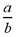, where a is called the numerator, b is called the denominator and b cannot equal 0 (since division by 0 is undefined).

# Reducing Fractions

Fractions can be reduced if the numerator and denominator have a greatest common divisor(gcd) greater than 1. The gcd refers to the largest possible integer that will divide evenly into each value. For example, gcd(6,18) = 6, since 6/6 = 1 and 18/6 =3. Six is the largest integer that will divide evenly into each number. If this example were a fraction, we would have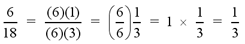Greatest Common Divisor / Least Common Multiple Calculator

# Examples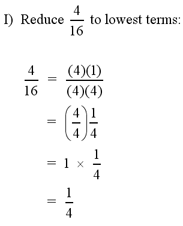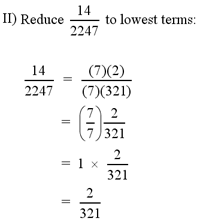# Converting Decimals to Fractions

Any rational number has a fractional representation. Decimals can be written as fractions if they are terminating or repeating. The following examples will show the step by step method of how to convert a decimal to a fraction.

# Examples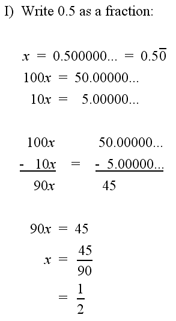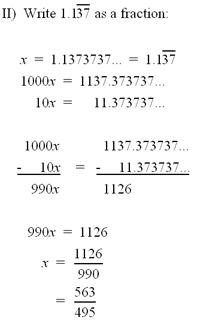Note: For a more complex example of decimal to fraction conversion, see question #1 in the Additional Examples section at the bottom of the page.

# Addition and Subtraction of Fractions

When adding or subtracting fractions, they must have the same denominator. If they do not have the same denomimator, we must find a common one for both. To do this, we first need to find the lowest common multiple of the two denominators. The lowest common multiple is the smallest number that both denominators will divide into evenly. For example, the lowest common multiple of 2 and 3 is 6.

Next, we must multiply each fraction by the proper integers so that they will have the same denominator. Continuing our example, if the two fractions are 1/2 and 1/3, we get: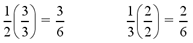Now that the two fractions have the same denominator, we can simply add or subtract them.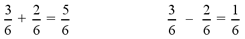# Examples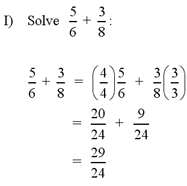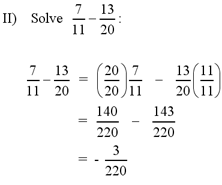# Improper Fractions and Mixed Numbers

Fractions can be written as improper fractions or mixed numbers.

Improper fractions (ex.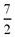) are fractions that have a numerator larger than their denominator.
Mixed numbers (ex.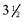) have an integer value followed by a proper fraction.

To convert from an improper fraction to a mixed number, we first divide the numerator by the denominator. The whole number becomes the integer in the mixed number. Any remainder from the divison is placed over the denominator and becomes the proper fraction.

To convert from a mixed number to an improper fraction, we first convert the integer to a fraction by multiply the integer value by the denominator in the proper fraction and placing it over the denominator. Add the resulting fraction to the proper fraction portion of the mixed number.

# Examples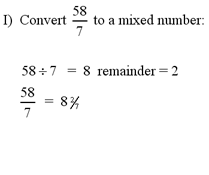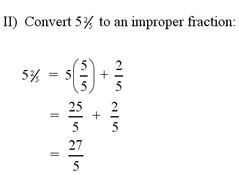# Multiplication and Division of Fractions

When multiplying two fractions, simply multiply the two numerators and multiply the two denominators. For example,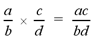When dividing two fractions, the first fraction must be multiplied by the reciprocal of the second fraction. For example,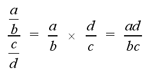# Examples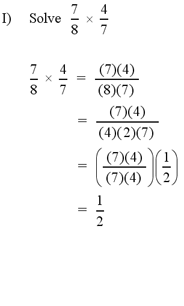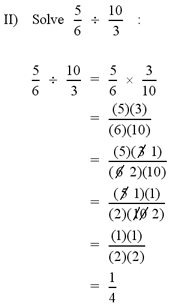1 | Example of decimal to fraction conversion

| Top of Page |

COURSE HOMEPAGES
MATH 1036
MATH 1037

FACULTY HOMEPAGES
Alex Karassev
Ted Chase